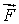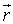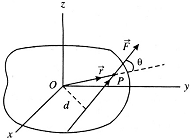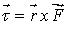# Physics Homework Help With Torque

## Torque Assignment Help

Consider a rigid body capable of rotating about the z-axis through O. Let a forceact on the body in the xy -plane at some point P whose position vector with respect to O is. If d is the perpendicular distance of the line of action of the force from the axis, then the torque acting on the body due to this force is defined as= Fd = Fr sin q

Torque is also called the moment of force and it is a measure of the turning effect of force about the given axis. The same force produces a larger turning effect, if its line of action is farther from the axis. Torque is actually a vector quantity having magnitude rF sinq and having direction along the axis of rotation. In vector notation, it is written asTorque is rotational analogue of force. A rigid body is a system of particles in which the distances between the particles are fixed.

Basic equation of Rotation

The relation t = Ia is the fundamental equation of rotation. It is the exact counterpart of the equation F = ma in linear motion. An unbalanced torque is necessary to give a body an angular acceleration just as an unbalanced force is required to give a body a linear acceleration. The quantities t, I and a play similar roles in angular motion as F, m and a play in translatory motion. Therefore for rotational motion we use

t = Ia

### Homework Help For Torque

Assignmenthelp.net provides best Online Assignment Help service in Physics for all standards. Our Tutor provide their high quality and optimized Tutorial help to fulfill all kind of need of Students.